# Triangular Pyramid Surface Area Calculator

Choose the measure you want to calculate and enter the required values in the triangular pyramid surface area calculator.

Give Us Feedback

## Triangular Pyramid Surface Area Calculator

The surface area of a triangular pyramid calculator can be used to find the base length, volume, lateral surface area, etc. The formula for each property appears at the side.

## What is a triangular pyramid?

A polyhedron having three triangular sides meeting at the apex on top and forming a triangular base at the bottom.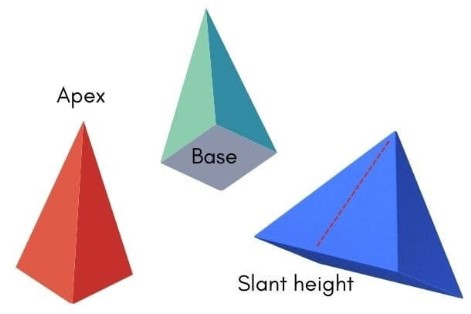A triangular pyramid has 6 edges and 4 vertices. A triangular pyramid can be either regular (equilateral) or non-regular (isosceles).

Below are some properties of a pyramid. These can be calculated through the triangular pyramid calculator.

### Surface Area - Definition

The surface area of a pyramid consists of the area of all the surfaces including the base.

The formula to calculate surface area is: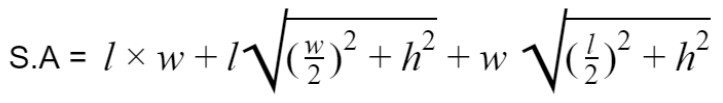Where,

• L = base length
• w = base width
• h = pyramid height

### Lateral Surface Area

It is also a type of surface area measurement but it excludes the base of the pyramid.

Its formula is: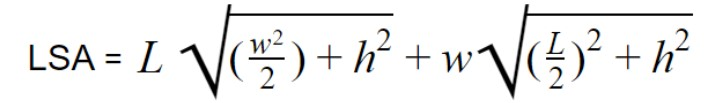Where,

• L = base length
• w = base width
• h = pyramid height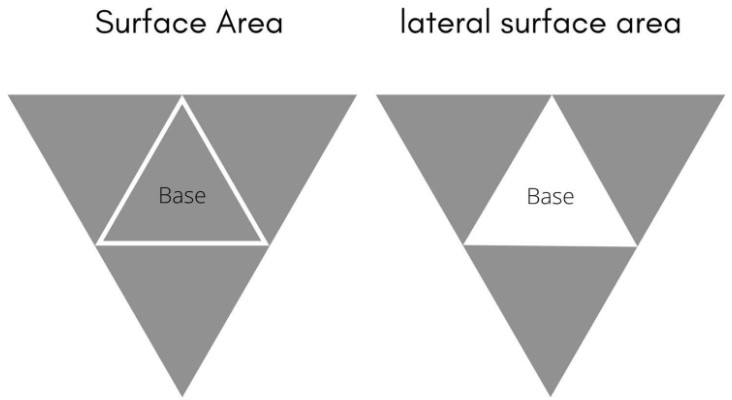### Volume

The total area occupied by a triangular pyramid in 3 dimensions (volume) is calculated by:

Volume = ( L x W x h )/ 3

In this equation:

• L stands for length
• W stands for width
• h stands for height

### Height

The distance from the center of the base to the apex is called the height of the pyramid. It is calculated by:

Height = 3v/lw

• V = volume
• L = base length
• w = base width

## How to Calculate Surface Area of a Triangular Pyramid?

Example:

For the given set of information, calculate surface area.

Base length = 6 cm

Base width  = 7cm

Height = 5cm

Solution:

Step 1: Write the formula.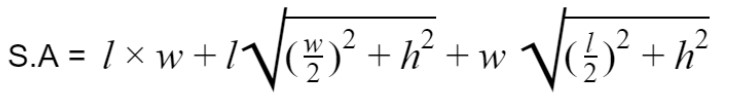Step 2: Put the values in the formula.

S.A = 6 * 7 + 6 √((7/2)2+52)+ 7√((6/2)2+52)

S.A = 6 * 7 + 36.62 + 40.8

S.A = 78.62 +40.8

S.A = 119.44

### Other Languages